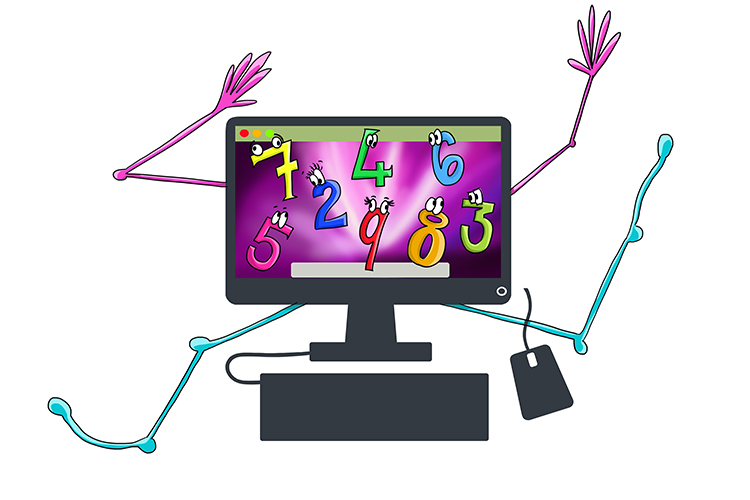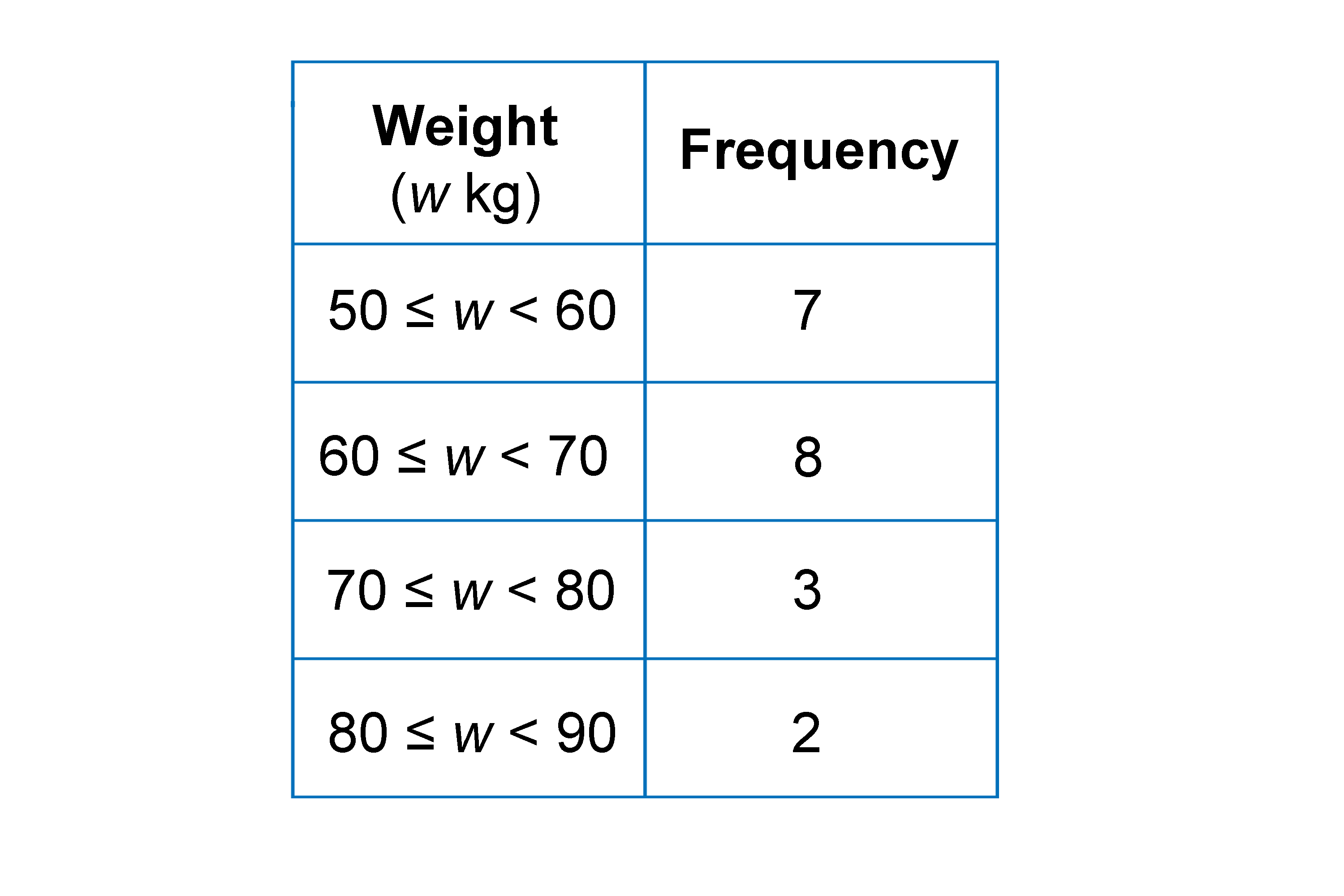# Random sampling

A method of sampling a population using an equal chance of being selected.

Random sampling means that each has the same chance of being selected.Random number generators are used in the lottery.

Equal chances of picking out a sample of, say, people from a population can be like picking a number out of a hat, giving you a random result.The lottery is a random selection of numbers.A computer can generate numbers randomly.

Example 1

The grouped frequency table shows information about the weights, in kilograms, of 20 students, chosen at random from Year 11.There are 300 students in Year 11. Work out an estimate for the number of students in Year 11 whose weight is between 50 kg and 60 kg.

300=20   (100% of the sample)

x=7

(see our section on percentages)

300/x=20/7

Therefore x=(300times7)/20

x=105